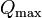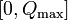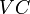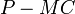# Determination of quantity supplied by firm in perfectly competitive market in the short run with increasing marginal cost

This is the development of a special case of determination of quantity supplied by firm in perfectly competitive market in the short run. The notation builds on notation used in that page.

## Recall of assumptions and setup

We do not recall all the definitions, but we do recall the key assumptions:

• The firm can choose to produce any quantity between$0$ and a maximum quantity$Q_{\max}$.
• The marginal cost$MC$ is defined at all quantities of production in$[0,Q_{\max}]$. Formally, it is the derivative of$VC$ with respect to$Q$, though at the endpoints$0$ and$Q_{\max}$, we take one-sided derivatives.
• For simplicity, we also assume that the$MC$ curve is continuous and is not highly oscillatory, i.e., we can break up the interval$[0,Q_\max]$ into finitely many pieces and the curve is either increasing or decreasing on each piece.

## Key general principle for profit maximization

The absolute (i.e., global) maximum for profit must occur either at one of these three points: zero quantity of production, the maximum quantity of production, or some local maximum at an intermediate quantity of production. In the last case, that must be a point where$P = MC$ and the$MC$ curve is rising.

## Description of case

In the simple case where marginal cost is an increasing function of quantity throughout the range of quantities that the firm can produce, the locally and globally optimal choice is as follows: keep increasing quantity produced until the marginal cost equals the market price. This is because as long as the marginal cost of production is less than the market price, the firm makes a profit by producing and selling at the market price. This is often stated as: the firm produces the quantity at which$P = MC$.

## Profit-maximizing choice

### Summary of choices

Case for relation between market price and marginal cost Quantity of production chosen to maximize profits Explanation in terms of formal mathematical analysis (see Determination of quantity supplied by firm in perfectly competitive market in the short run#Formal mathematical analysis)
The market price is less than the marginal cost for all quantities, i.e., even starting with zero quantity Zero. In other words, the firm stays idle and waits for the market price to rise. In this case, the profit function is decreasing throughout the interval$[0,Q_\max]$ (because the derivative$P - MC$ is negative) and the maximum profit can thus be attained by producing nothing.
There is a (unique) quantity the firm can produce at which the marginal cost equals the market price and is about to exceed it. Produce at the quantity (i.e., the quantity where$P = MC$). For smaller quantities of production, the profit function is increasing, because$P - MC$ is positive. For larger quantities of production, the profit function is decreasing, because$P - MC$ is negative. Thus, the quantity at which$P = MC$ gives the unique local and absolute maximum for the firm.
Even at the maximum quantity that the firm can produce, the market price is more than the marginal cost. Produce at the maximum quantity. The profit function is increasing on the entire interval$[0,Q_\max]$, because$P - MC$ is positive throughout. So the maximum profit is attained at the maximum quantity of production.

### Key price and quantity values

• The reservation price of the firm is the marginal cost at quantity zero, i.e., the point at which the marginal cost curve begins on the price axis.
• There is also a maximum price of interest: the marginal cost at maximum quantity of production. For prices higher than this, the firm still produces the same quantity$Q_\max$. In other words, its strategy is price-insensitive beyond the marginal cost at$Q_\max$.

### Nature of short run supply curve

The graphical interpretation of this is that the firm's short run supply curve coincides with its marginal cost curve for any price ranging from the reservation price to the marginal cost at maximum quantity of production. The part of the short run supply curve for prices between 0 and the reservation price is a vertical line segment and the part of the short run supply curve for prices beyond the maximum marginal cost is a vertical line.

## Case of reservation price zero

The figure below illustrates a situation where the reservation price is zero, i.e., for any positive price, the firm will produce a nonzero quantity of the good:

The reservation price in this case is zero. Note that if there is a maximum quantity of production$Q_\max$, then the short run supply curve for prices beyond the marginal cost at$Q_\max$ is a vertical line with quantity coordinate$Q_\max$. (Picture needs to be updated)

### Case of positive reservation price

The next figure illustrates a situation where the reservation price is positive, because even the smallest possible value of marginal cost is positive, i.e., the marginal cost does not start from zero. What this means is that if the market price of the good is less than the reservation price, the firm will stay idle, i.e., will not produce anything:

The part of the short run supply curve for price between 0 and the reservation price is a vertical line segment along the price axis. The part of the short run supply curve for price greater than the marginal cost at maximum quantity of production is a vertical line with quantity coordinate$Q_\max$.